Test: Special Classes of Random Processes

# Test: Special Classes of Random Processes

Test Description

## 8 Questions MCQ Test Communication System | Test: Special Classes of Random Processes

Test: Special Classes of Random Processes for Electronics and Communication Engineering (ECE) 2023 is part of Communication System preparation. The Test: Special Classes of Random Processes questions and answers have been prepared according to the Electronics and Communication Engineering (ECE) exam syllabus.The Test: Special Classes of Random Processes MCQs are made for Electronics and Communication Engineering (ECE) 2023 Exam. Find important definitions, questions, notes, meanings, examples, exercises, MCQs and online tests for Test: Special Classes of Random Processes below.
Solutions of Test: Special Classes of Random Processes questions in English are available as part of our Communication System for Electronics and Communication Engineering (ECE) & Test: Special Classes of Random Processes solutions in Hindi for Communication System course. Download more important topics, notes, lectures and mock test series for Electronics and Communication Engineering (ECE) Exam by signing up for free. Attempt Test: Special Classes of Random Processes | 8 questions in 30 minutes | Mock test for Electronics and Communication Engineering (ECE) preparation | Free important questions MCQ to study Communication System for Electronics and Communication Engineering (ECE) Exam | Download free PDF with solutions
 1 Crore+ students have signed up on EduRev. Have you?
Test: Special Classes of Random Processes - Question 1

### Let U and V be two independent zero mean Gaussian random variables of variances 1/4 and 1/9 respectively. The probability P(3V ≥ 2U) is

Detailed Solution for Test: Special Classes of Random Processes - Question 1

U and V are two independent zero mean and Gaussian.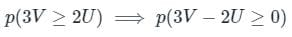let z = 3V - 2U

U and V are Gaussian then their linear transformation Z is also Gaussian

than E[z] = E[3V - 2U]=3E[V] - 2E[U]=0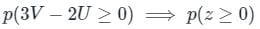if z is gaussian and zero mean then its probability for greater than zero is 0.5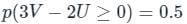Option C is the correct answer.

Test: Special Classes of Random Processes - Question 2

### A device has 200 Ω equivalent noise resistance, 300 Ω input resistor, and the bandwidth of the amplifier is 6 MHz. If the operating temperature of the amplifier is 290° K, the noise voltage at the input of a television RF amplifier will be nearly

Detailed Solution for Test: Special Classes of Random Processes - Question 2

Concept:

The noise voltage at the input of the RF amplifier is given by: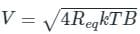Where,

Equivalent resistance (Req) = RNoise + Rin

Boltzmann constant (k) = 1.38 × 10-23

T: Operating temperature.

B: Bandwidth of amplifier.

Calculation:

Given Equivalent resistance (Req) = 200 + 300 = 500 Ω

B = 6 MHz and T = 290 0K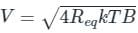Putting on the respective values, we get: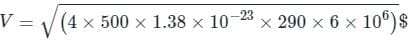V = 6.92 μV

Test: Special Classes of Random Processes - Question 3

### Which method is much better and efficient?

Detailed Solution for Test: Special Classes of Random Processes - Question 3

Vector quantization will always equal or exceed the performance of scalar quantization.

Test: Special Classes of Random Processes - Question 4

Which reduces the dynamic range of quantization noise in PCM?

Detailed Solution for Test: Special Classes of Random Processes - Question 4

Adaptive quantizer reduces the dynamic range of quantization noise in PCM and DPCM.

Test: Special Classes of Random Processes - Question 5

Gaussian process is a

Detailed Solution for Test: Special Classes of Random Processes - Question 5

If Gaussian process is a wide sense stationary process then it will also be strict sense stationary process.

Test: Special Classes of Random Processes - Question 6

Air craft of Jet Airways at Ahmedabad airport arrive according to a Poisson process at a rate of 12 per hour. All aircraft are handled by one air traffic controller. If the controller takes a 2 – minute coffee break, what is the probability that he will miss one or more arriving aircraft?

Detailed Solution for Test: Special Classes of Random Processes - Question 6

P (miss/or more aircraft) = 1 – P(miss 0) = 1 – P(0 arrive).

Test: Special Classes of Random Processes - Question 7

A random process is defined by X(t) + A where A is continuous random variable uniformly distributed on (0,1). The auto correlation function and mean of the process is

Detailed Solution for Test: Special Classes of Random Processes - Question 7

E[X(t)X(t + t)] = 1/3 and E[X(t)] = 1/2 respectively.

Test: Special Classes of Random Processes - Question 8

The auto correlation function of a stationary ergodic random process is shown below.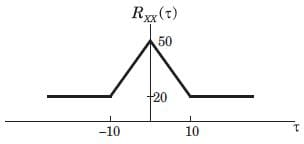What is the value of variance?

Detailed Solution for Test: Special Classes of Random Processes - Question 8

Here X = 0, y = 0, Rxx(0) = 5, Ryy(0) = 10. The only value that satisfies all the given conditions is 30.

## Communication System

37 docs|22 tests
Information about Test: Special Classes of Random Processes Page
In this test you can find the Exam questions for Test: Special Classes of Random Processes solved & explained in the simplest way possible. Besides giving Questions and answers for Test: Special Classes of Random Processes, EduRev gives you an ample number of Online tests for practice

## Communication System

37 docs|22 tests(Scan QR code)